Learn from home

The teachersA right triangle has a right angle (90º) and two acute angles.The hypotenuse is the side opposite the right angle and is the largest side of the triangle.

The legs or catheti (singular: cathetus) are the sides opposite the acute angles and are the shorter sides of the triangle.

### Pythagorean Theorem

In a right triangle, the square of the hypotenuse equals the sum of the squares of the legs.Determine whether the following triangle is a right triangle:The legs of a right triangle measure at 3 m and 4 m. What is the length of the hypotenuse?The hypotenuse of a right triangle is 5 m and one of its legs is 3 m. What is the length of the other leg?### Problems

A ladder 10 m long is leaning against a wall. The foot of the ladder is 6 m from the base of the wall. At what height does the ladder rest on the wall?Find the area of the equilateral triangle:Find the diagonal of the square: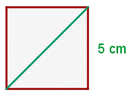Find the diagonal of the rectangle: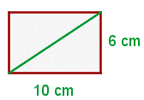Find the perimeter and area of the right trapezoid: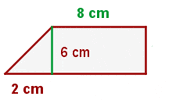P = 8 + 6 + 12 + 6.32 = 32.32 cmThe perimeter of an isosceles trapezoid is 110 m, the bases are 40 and 30 m respectively. Calculate the length of the non-parallel sides and the area.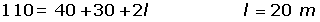Find the area of the regular pentagon: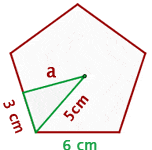Calculate the area of the square inscribed in a circle with a circumference of 18.84 m.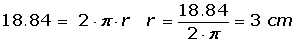In a circle a chord of 48 cm is 7 cm from the center. Calculate the area of the circle.Did you like the article?(1 votes, average: 5.00 out of 5)Loading...

Emma

I am passionate about travelling and currently live and work in Paris. I like to spend my time reading, gardening, running, learning languages and exploring new places.

Did you like
this resource?

Bravo!